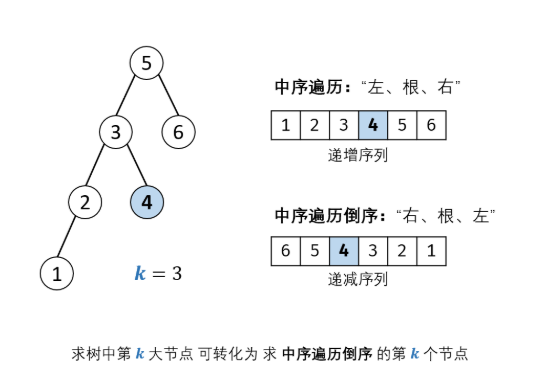## Title description

Given a binary search tree, please find the k-th largest node.

## Example questions

Example 1:

Input: root = [3,1,4, null, 2], k = 1
3
/ \
1 4
\
2
Output: 4
Example 2:

Input: the root = [5,3,6,2,4, null, null,. 1], K =. 3
. 5
/ \
. 3. 6
/ \
2 4
/
. 1
Output: 4

## Problem-solving ideasAnalyzing the problem, we can find that the mid-order traversal of the binary search tree (left subtree, root node, right subtree) is a monotonically increasing sequence . Finding the k-th largest node of the binary search tree is equivalent to finding the binary search tree. The k-th node in the reverse order of the middle-order traversal , and the reverse-order traversal of the binary search tree (right subtree, root node, left subtree) is a monotonically decreasing sequence . Because the problem is known as a binary search tree, it is sorted by default. If the k-th largest node of the binary tree is required? So how to do it? The usual way is to use the order of the traversal in the binary tree, store the traversed values ​​in the array, and then output the penultimate k-th element. Of course, there is another classic method that uses priority queues to implement large and small top heaps.

The priority queue defaults to a large top heap, which meets the requirements of the topic, so we use the depth-first search method to add the values ​​of all nodes in the tree to the priority queue (default large top heap), and then pop the first k-1 elements in the priority queue , And finally, the head element is evaluated.

## Program source code

Recursive

```/**
* Definition for a binary tree node.
* struct TreeNode {
*     int val;
*     TreeNode *left;
*     TreeNode *right;
*     TreeNode(int x) : val(x), left(NULL), right(NULL) {}
* };
*/
class Solution {
public:
int cnt = 0;
int res_num = 0;
int kthLargest(TreeNode* root, int k) {
dfs(root,k);
return res_num;
}
void dfs(TreeNode* root, int k)
{
if(root == nullptr) return ;
dfs(root->right, k);
cnt++;
if(cnt == k)
res_num = root->val;
dfs(root->left, k);

}
};```

Iteration (stack)

```/**
* Definition for a binary tree node.
* struct TreeNode {
*     int val;
*     TreeNode *left;
*     TreeNode *right;
*     TreeNode(int x) : val(x), left(NULL), right(NULL) {}
* };
*/
class Solution {
public:
int cnt = 0; //维护一个计数器
int kthLargest(TreeNode* root, int k) {
if(root == nullptr) return 0;
TreeNode* p = root;
stack<TreeNode*> s;
s.push(p);
while(p || !s.empty())
{
while(p)
{
s.push(p);
p = p->right;
}
p = s.top();
s.pop();
cnt++;
if(cnt == k) return p->val;
p = p->left;
}
return 0;
}
};```

Large top heap (priority queue)

```/**
* Definition for a binary tree node.
* struct TreeNode {
*     int val;
*     TreeNode *left;
*     TreeNode *right;
*     TreeNode(int x) : val(x), left(NULL), right(NULL) {}
* };
*/
class Solution {
public:
priority_queue<int> prior_que;
void dfs(TreeNode* root)
{
prior_que.push(root->val);
if(root->left != nullptr) dfs(root->left);
if(root->right != nullptr) dfs(root->right);
}
int kthLargest(TreeNode* root, int k) {
dfs(root);
while(--k)
{
prior_que.pop();
}
return prior_que.top();
};```

Binary tree to find the k-th largest node in the penultimate (non-binary search tree, ie unordered)

```/**
* Definition for a binary tree node.
* struct TreeNode {
*     int val;
*     TreeNode *left;
*     TreeNode *right;
*     TreeNode(int x) : val(x), left(NULL), right(NULL) {}
* };
*/
class Solution {
public:
int kthLargest(TreeNode* root, int k) {

vector<int> res;
stack<TreeNode*> s;
TreeNode* p;
p = root;
while(p!=NULL || !s.empty())
{
while(p != NULL)
{
s.push(p);
p=p->left;
}
if(!s.empty())
{
p = s.top();
s.pop();
res.push_back(p->val);
p = p->right;
}
}
return res[res.size() - k];
}
};```

### Guess you like

Origin www.cnblogs.com/wzw0625/p/12677838.html
Recommended
Ranking
Daily